# Assignments

1. Electrical costs
The data for this assignment are listed in the Excel file Electricity costs.
A manufacturer has recorded its costs of electricity and the total number of hours of machine time for each of 52 weeks. Estimate the fixed and variable electricity costs.

Solution.
Use Excel and find that the equation of the regression line is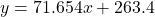, wheredenotes the hours of machine time and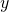the costs. This equation may be interpreted such that 263.4 denotes the fixed costs (zero hours electricity) and 71.654 the variable costs (for each unit of electricity).

Solution

2. Effects boat price
The data for this assignment are listed in the Excel file Effects boat price.
Millions of boats are registered in the United States. As is the case with automobiles, there is an active used-boat market. Many of the boats purchased require bank financing, and, as a result, it is important for financial institutions to be capable of accurately estimating the price of boats. One variable that affects the price is the number of hours the engine has been run. To determine the effect of the hours on the price, a financial analyst recorded the price (in 1000 dollars) of a sample of 24-foot Sea Ray cruisers (from 2007, one of the most popular boats) and the number of hours they had been run.
a. Determine the regression equation of the regression line.
c. What is the price of the boat when it has been run zero hours and comment its usefulness.
d. Estimate the price of the boat when it has been run 1380 hours.
e. Explain how you could predict with 99% confidence the price of a 1999  24-foot Sea Ray cruiser with 500 hours of engine use (no actual calculations are required).

Solution.
a. The regression line can be found by using the Data Analysis option of Excel: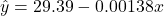. If the Data Analysis option is not used, then we can compute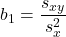, where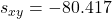and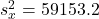and thus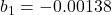and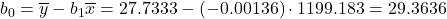.
b. The price of each additional hour is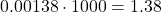.
c. The price of the boat when it has been run zero hours would have been 29,390. However, this amount is meaningless because there are no data available for so few hours.
d. The price for a boat when it has been run 1380 hours is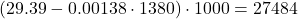.
e. Use the formula of the prediction interval, see Keller, p. 640.

3. Study time
The data for this assignment are listed in the Excel file Study time.
Are the marks one receives in a course related to the amount of time spent studying the subject? To analyze this mysterious possibility, a student took a random sample of 10 students who had enrolled in a statistics class last semester. He asked to report his or her mark in the course and the total number of hours spent studying statistics. These data are listed  here.

Use Excel to answer the following questions and explain how you did it.
a. Calculate the coefficient of correlation. What does the result tell you?
b. Determine the least squares line.
c. What do the statistics calculated above tell you about the relationship between marks and study time?
d. According to these results, how much time do you need to spend studying statistics to expect a mark of 100? Explain why this result is not useful.

Solution.
a. You can do it by hand, but this takes a lot of computation. Excel can do the job for you: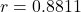. This result tells us that there is a strong positive relation between Marks and Time Spent: the more time you spend on studying, the higher your expected mark.
b. Again Excel is used to find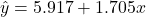.
c. The line shows again the strong positive linear relationship between marks and study time: for each additional hour of study time marks increase on average by 1.705.
d. Solvefrom the equation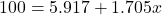and find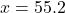hours. This result is not really useful since there are no observations around the mark 100.

0